Latest Banking jobs   »

# Quantitative Aptitude Quiz For Bank Foundation 2023 – 07th April

Directions (1-5): In each of the following questions two equations are given. Solve these equations and give answer:
(a) if x≥y, i.e. x is greater than or equal to y
(b) if x>y, i.e. x is greater than y
(c) if x≤y, i.e. x is less than or equal to y
(d) if x<y, i.e. x is less than y
(e) x=y or no relation can be established between x and y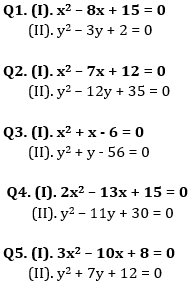Directions (6-10): In each of these questions, two equation (I) and (II) are given. You have to solve both the equations and give answer
(a) If x>y
(b) If x≥y
(c) If x<y
(d) If x≤y
(e) If x = y or no relation can be established between x and y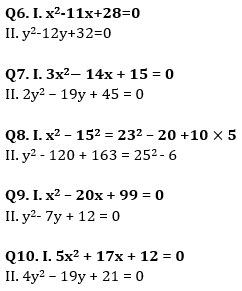Directions (11-15): In each of the following questions two equations are given. Solve these equations and give answer:
(a) if x≥y, i.e. x is greater than or equal to y
(b) if x>y, i.e. x is greater than y
(c) if x≤y, i.e. x is less than or equal to y
(d) if x<y, i.e. x is less than y
(e) x=y or no relation can be established between x and y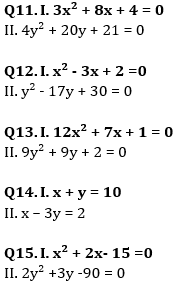Solutions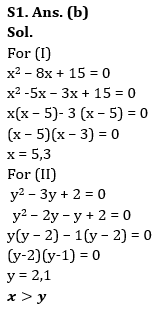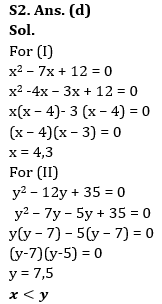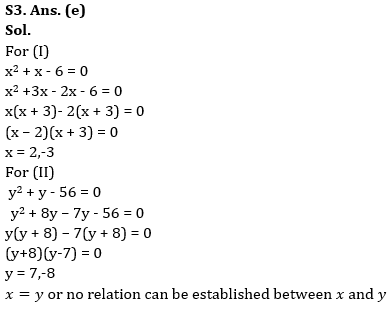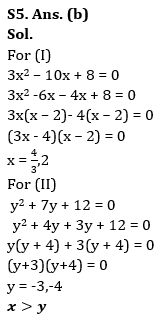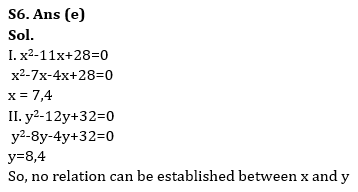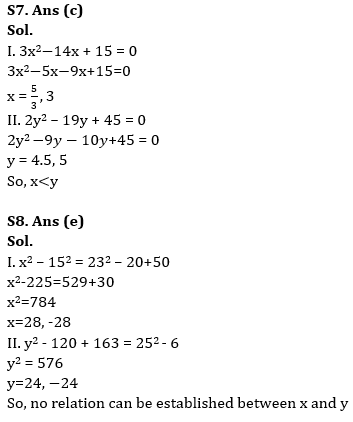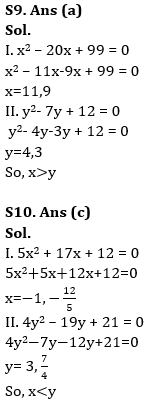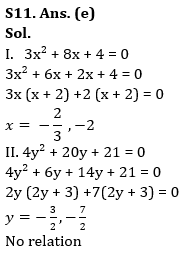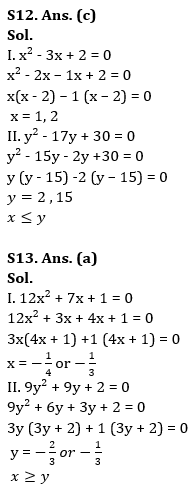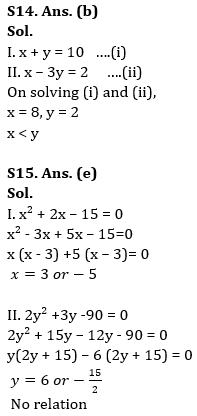.                                                                                                                                   .

## FAQs

### What is the selection process of the Bank Clerk?

The selection process of the Bank Clerk is Prelims & Mains.

#### Congratulations!Union Budget 2023-24: Free PDF